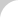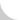The Complete Guide to Chain# 2.1.1 Elastic Stretch, Plastic Deformation, and Breakage

Tensile Strength

How will the chain behave when it is subject to tensile loading? There is a standard test to determine the tensile strength of a chain. Here's how it works: The manufacturer takes a new, five-link-or-longer power transmission chain and firmly affixes both ends to the jigs (Figure 2.1). Now a load or tension is applied and measurements are taken until the chain breaks (JIS B 1801-1990).

Chain Elongation

As a chain is subject to increasing stress or load, it becomes longer. This relationship can be graphed (Figure 2.2). The vertical axis shows increasing stress or load, and the horizontal axis shows increasing strain or elongation. In this stress-strain graph, each point represents the following:

• O-A:  elastic region
• A:  limit of proportionality for chains; there is not an obvious declining point, as in mild steel
• A-C:  plastic deformation
• B:  maximum tension point
• C:  actual breakageFigure 2.1 Typical Chain in Tensile TestFigure 2.2 Stress-Strain GraphFigure 2.3 Tensile Strength

Reporting Tensile Strength

Point B, shown in Figure 2.2, the maximum tension point, is also called the ultimate tensile strength. In some cases, point B will come at the same time as point C. After breaking a number of chains, a tensile strength graph shows a normal distribution (Figure 2.3).

The average load in Figure 2.3 is called the average tensile strength, and the lowest value, which is determined after statistically examining the results, is called the minimum tensile strength. JIS (Japanese Industrial Standard) also regulates minimum tensile strength, but is much lower than any manufacturer's tensile strength listed in their catalogs.

"Maximum allowable load," shown in some manufacturer's catalogs, is based on the fatigue limit (see Basics Section 2.2.2). This value is much lower than point A. Furthermore, in the case of power transmission chain, point A is usually 70 percent of the ultimate tensile strength (point B). If the chain receives greater tension than point A, plastic deformation will occur, and the chain will be nonfunctional.

Using Tensile Strength Information

For the sake of safety, you should never subject chains to tension greater than half the average tensile strength -not even once. If the chain is inadvertently loaded that high, you should change the whole chain set. If the chain is repeatedly subject to loads greater than the maximum allowable load, fatigue failure may result.

When you see tensile strength graphs or stress-strain graphs, you should be aware of the following facts:

1. Every manufacture shows the average tensile strength in its catalog, but it is not unusual to find that the value listed may have been developed with sales in mind. Therefore, when comparing chains from different manufactures, check the minimum tensile strength.
2. In addition to the tensile strength, the most important fact about a stress-strain graph is the value of stretch at the time of breakage. If the chain's tensile strength is higher and the capacity to stretch is greater, the chain can absorb more energy before it breaks. This means the chain won't be easily broken even if it receives unexpected shock load. (In Figure 2.2, the cross-hatched area is the value of energy that the chain can absorb before it breaks.)

Elastic Elongation

Another important characteristic in practice is how much elastic elongation the chain will undergo when it is subjected to tension. When you use chains for elevators on stage, if there is a difference between the stage floor and the elevator platform, the dancers will trip on it. In an elevator parking garage, it is necessary to lower cars down to the entrance within a small difference in the level. Therefore, it is important to anticipate how long the chain's elastic stretch will be. Figure 2.4 shows elasticity/stretch for power transmission roller chains.